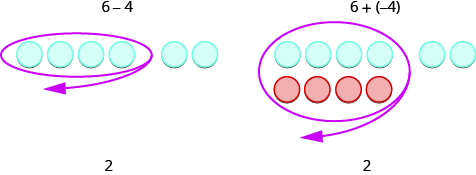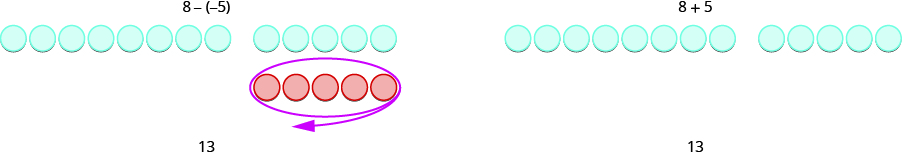## Simplifying and Evaluating Expressions With Integers

### Learning Outcomes

• Simplify expressions using subtraction of integers
• Evaluate variable expressions with subtraction of integers

Now that you have seen subtraction modeled with color counters, we can move on to performing subtraction of integers without the models.

• Subtract $-23 - 7$. Think: We start with $23$ negative counters. We have to subtract $7$ positives, but there are no positives to take away. So we add $7$ neutral pairs to get the $7$ positives. Now we take away the $7$ positives. So what’s left? We have the original $23$ negatives plus $7$ more negatives from the neutral pair. The result is $30$ negatives. $-23 - 7=-30$ Notice, that to subtract $\text{7,}$ we added $7$ negatives.
• Subtract $30-\left(-12\right)$. Think: We start with $30$ positives. We have to subtract $12$ negatives, but there are no negatives to take away. So we add $12$ neutral pairs to the $30$ positives. Now we take away the $12$ negatives. What’s left? We have the original $30$ positives plus $12$ more positives from the neutral pairs. The result is $42$ positives. $30-\left(-12\right)=42$ Notice that to subtract $-12$, we added $12$.

While we may not always use the counters, especially when we work with large numbers, practicing with them first gave us a concrete way to apply the concept, so that we can visualize and remember how to do the subtraction without the counters.

Have you noticed that subtraction of signed numbers can be done by adding the opposite? You will often see the idea, the Subtraction Property, written as follows:

### Subtraction Property

Subtracting a number is the same as adding it’s opposite.

$a-b=a+(-b)$

Look at these two examples.We see that $6 - 4$ gives the same answer as $6+\left(-4\right)$.

Of course, when we have a subtraction problem that has only positive numbers, like the first example, we just do the subtraction. We already knew how to subtract $6 - 4$ long ago. But knowing that $6 - 4$ gives the same answer as $6+\left(-4\right)$ helps when we are subtracting negative numbers.

### example

Simplify:

1. $13 - 8\text{ and }13+\left(-8\right)$
2. $-17 - 9\text{ and }-17+\left(-9\right)$

Solution:

 1. $13 - 8$ and $13+\left(-8\right)$ Subtract to simplify. $13 - 8=5$ Add to simplify. $13+\left(-8\right)=5$ Subtracting $8$ from $13$ is the same as adding $−8$ to $13$.
 2. $-17 - 9$ and $-17+\left(-9\right)$ Subtract to simplify. $-17 - 9=-26$ Add to simplify. $-17+\left(-9\right)=-26$ Subtracting $9$ from $−17$ is the same as adding $−9$ to $−17$.

Now you can try a similar problem.

### try it

Now look what happens when we subtract a negative.We see that $8-\left(-5\right)$ gives the same result as $8+5$. Subtracting a negative number is like adding a positive. In the next example, we will see more examples of this concept.

### example

Simplify:

1. $9-\left(-15\right)\text{ and }9+15$
2. $-7-\left(-4\right)\text{ and }-7+4$

Now you can try a similar problem.

### try it

The table below summarizes the four different scenarios we encountered in the previous examples, and how you would use counters to simplify.

 $5 - 3$ $-5-\left(-3\right)$ $2$ $-2$ $2$ positives $2$ negatives When there would be enough counters of the color to take away, subtract. $-5 - 3$ $5-\left(-3\right)$ $-8$ $8$ $5$ negatives, want to subtract $3$ positives $5$ positives, want to subtract $3$ negatives need neutral pairs need neutral pairs When there would not be enough of the counters to take away, add neutral pairs.

In our next example we show how to subtract a negative with two digit numbers.

### example

Simplify: $-74-\left(-58\right)$.

Now you can try a similar problem.

### try it

In the following video we show another example of subtracting two digit integers.

Now let’s increase the complexity of the examples a little bit. We will use the order of operations to simplify terms in parentheses before we subtract from left to right.

### example

Simplify: $7-\left(-4 - 3\right)-9$

Now you try it.

### try it

Watch the following video to see more examples of simplifying integer expressions that involve subtraction.

Now we will add another operation to an expression. Because multiplication and division come before addition and subtraction, we will multiply, then subtract.

### example

Simplify: $3\cdot 7 - 4\cdot 7 - 5\cdot 8$.

Now you try.

### try it

Watch the following video to see another example of simplifying an integer expression involving multiplication and subtraction.

### Evaluate Variable Expressions with Integers

Now we’ll practice evaluating expressions that involve subtracting negative numbers as well as positive numbers.

### example

Evaluate $x - 4\text{ when}$

1. $x=3$
2. $x=-6$.

Now you try.

### try it

In the next example, we will subtract a positive and a negative.

### example

Evaluate $20-z\text{ when}$

1. $z=12$
2. $z=-12$

Now you try.

## Contribute!

Did you have an idea for improving this content? We’d love your input.